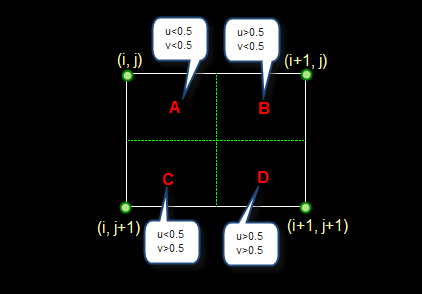# 图像处理

## 第二章 数字图像基础

### 人类的视觉感知系统

• 视觉是人类最高级的感知器官

• 然而人类感知只限于电磁波谱的视觉波段，成像机器则可以覆盖几乎全部电磁波谱

• 研究图像处理首先要了解人类的视觉感知系统

### 图像感知和获取

• 单个传感器

优点：精度高、成本低

缺点：速度慢

• 带状传感器

优点：可获得物体截面图像、成本较低

缺点：速度慢

• 传感器阵列

各类图像都是由“照射”源和形成图像的“场景”元素对光能的反射或吸收相结合而产生的。

#### 简单的图像形成模型

1. 入射到观察场景的光源总量$i(x,y)$

2. 场景中物体反射光的总量$r(x,y)$

$i(x,y)$的性质取决于照射源，而$r(x,y)$取决于成像物体的特性。反射分量$r(x,y)$限制在0（全吸收）和1（全反射）之间。

$i(x,y)$的某些典型值：在晴朗的白天，太阳在地球表面产生的照度超过90000lm/m2。在有云的情况下，这个数值下降到1000lm/m2。在晴朗的夜晚，满月情况下大约为0.1lm/m2的照度。

$r(x,y)$的某些典型值：黑天鹅绒为0.01，不锈钢为0.65，白色墙为0.80

Intrinsic image decomposition 本征图像分解

#### 图像的数字化

• 取样：

将在空间上连续分布的图像转换成离散的取样点（即像素）集的操作。由于图像是二维分布的信息，所以取样是在x轴和y轴两个方向上进行。

取样时注意：取样间隔的选取。取样间隔取得不合适除了画面出现马赛克之外，还会发生频率的混合现象。

• 量化：

将各个像素所含的明暗信息离散化后用数字来表示，称为图像的量化。一般的量化值用整数表示。充分考虑到人眼的识别能力后，目前非特殊用途的图像均为8 bit量化，即用0～255描述“黑～白”

#### 空间和灰度级分辨率

• 在取样时，若横向的像素数（列数）为M ，纵向的像素数（行数）为N，则图像总像素数为 M * N 个像素。 一般来说，采样间隔越大，所得图像像素数越少，空间分辨率低，质量差，严重时出现马赛克效应；采样间隔越小，所得图像像素数越多，空间分辨率高，图像质量好，但数据量大。

• 量化等级越多，所得图像层次越丰富，灰度分辨率高，图像质量好，但数据量大

• 量化等级越少，会出现假轮廓现象，图像质量变差，但数据量小

#### 像素之间的邻域关系

4邻域：

• 像素$p(x,y)$的4邻域是$(x+1,y),(x-1,y),(x,y+1),(x,y-1)$

• 用$N_4(p)$表示像素p的4邻域

D邻域：

• 像素$p(x,y)$的D邻域是对角上的点$(x+1,y+1),(x+1,y-1),(x-1,y+1),(x-1,y-1)$

• 用$N_D(p)$表示像素p的D邻域

8邻域：

• $N_8(p)=N_4(p)+N_D(p)$

#### 图像的放大与缩小

• 最近邻

设i+u, j+v(i, j为正整数， u, v为大于零小于1的小数，下同)为待求像素坐标，则待求像素灰度的值 f(i+u, j+v)。

如果(i+u, j+v)落在A区，即u<0.5, v<0.5，则将左上角像素的灰度值赋给待求像素，同理，落在B区则赋予右上角的像素灰度值，落在C区则赋予左下角像素的灰度值，落在D区则赋予右下角像素的灰度值。最邻近元法计算量较小，但可能会造成插值生成的图像灰度上的不连续，在灰度变化的地方可能出现明显的锯齿状

• 双线性插值

简明版

#### 彩色模型

CIE(国际照明委员会)在进行大量的色彩测试实验的基础上提出了一系列的颜色模型：

• RGB模型：红、绿、蓝 三基色混合

• HSI模型：色度(H, Hue)、饱和度(S, Saturation)、亮度(I, Intensity)

HSI彩色模型比较适合于人用色调、饱和度、亮度描述被观察物体颜色的解释，对于开发基于彩色描述的图像处理方法是一个理性的工具。• H表示色调，用 角度 表示。 反映了该颜色最接近什么样的光谱波长。 0度为红色，逆时针120度为绿色，顺时针120度为蓝色。

• S表示饱和度，饱和度参数是色环的原点到彩色点的半径长度。在环的外围圆周是纯的或饱和的颜色，其饱和度值为1。在中心是中性（灰）色，即饱和度为0。

• I表示光照强度或亮度，它确定了像素的整体亮度，而不管其颜色是什么。

• YUV模型：亮度(Y)、色度(UV)

• YCbCr模型：亮度(Y)、色度(CbCr)

CMYK色系：用于印刷行业，是一种减色系统，将从白光中滤出三种原色之后获得的颜色作为表色系的三原色CMY

C (cyan)：青色/蓝绿色，从白色中滤去红色。

M (magenta)：品红，从白色中滤去绿色。

Y (yellow)：黄色，从白色中滤去蓝色。

• 最大值法：将输入图像中的每个像素的R、G、B分量值的最大者赋给输出图像中对应像素的R、G、B分量的方法。用公式可表示为：

$g_R(x,y)=g_G(x,y)=g_B(x,y)=max(f_R(x,y),f_G(x,y),f_B(x,y))$

• 平均值法：将输入图像中的每个像素的R、G、B分量的算术平均值赋给输出图像中对应像素的R、G、B分量的方法。用公式可表示为：

$g_R(x,y)=g_G(x,y)=g_B(x,y)=(f_R(x,y)+f_G(x,y)+f_B(x,y))/3$

• 加权平均值法：人眼对绿光的亮度感觉仅次于白光，是三基色中最亮的，红光次之，蓝光最低。相关研究表明，当$\omega_G$=0.587、$\omega_R$=0.299、$\omega_B$=0.114时，得到的灰度化图像较合理，此时灰度化公式就变为：

$g_R(x,y)=g_G(x,y)=g_B(x,y)=0.299\cdot f_R(x,y)+0.587\cdot f_G(x,y)+0.114\cdot f_B(x,y)$

preccrep

2021-11-18

###### Updated on

2021-11-18

You need to set install_url to use ShareThis. Please set it in _config.yml.
You forgot to set the shortname for Disqus. Please set it in _config.yml.
You need to set client_id and slot_id to show this AD unit. Please set it in _config.yml.